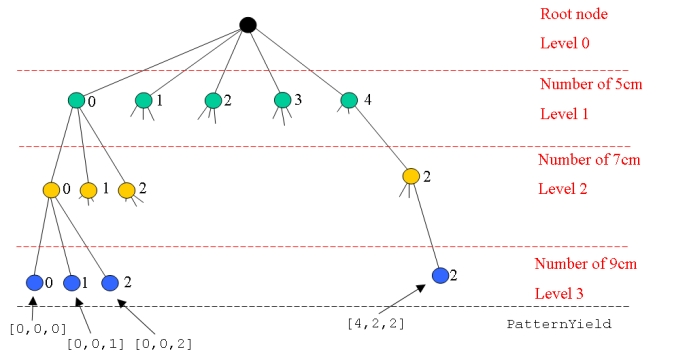# Difference: DepthFirstSearch (3 vs. 4)

Line: 1 to 1

 META TOPICPARENT name="SetPartitioning"

# Depth-First Search for Enumeration

Line: 12 to 12

```reset;

```
Changed:
<
<
model cutting_stock.mod; data cutting_stock.dat;
>
>
model cutting_stock_col.mod; data cutting_stock_col.dat;

param TotalLength; param Length {ROLLS} >= 0;

data;

param TotalLength := 20;

param Length := A 5 B 7 C 9 ;

model;

reset data PatternYield, PATTERNS; # Remove any pattern data, we will find it automatically

# Search the possibilities for cutting patterns

Changed:
<
<
param MinLength := min {i in ITEMS} Length[i]; # Find the minimum length of all the items param MaxPossible {i in ITEMS} >= 0, integer # Find the maximum number of each length item
>
>
param MinLength := min {i in ROLLS} Length[i]; # Find the minimum length of all the ROLLS param MaxPossible {i in ROLLS} >= 0, integer # Find the maximum number of each length item
:= TotalLength div Length[i]; # that can be cut from a roll

# The current product being considered

Changed:
<
<
param current symbolic within ITEMS;
>
>
param current symbolic within ROLLS;
# The number of each product being cut
Changed:
<
<
param number {ITEMS} >= 0, integer, default 0;
>
>
param number {ROLLS} >= 0, integer, default 0;
# Are we still searching? param stillSearching binary;
Line: 33 to 48
param newPattern > 0, integer;

let PATTERNS := {};

Changed:
<
<
# Start with the first product and no items being cut let current := first(ITEMS);
>
>
# Start with the first product and no ROLLS being cut let current := first(ROLLS);
let number[current] := 0; let stillSearching := 1; repeat { display current, number;
Changed:
<
<
if ( current <> last(ITEMS) ) and
>
>
if ( current <> last(ROLLS) ) and
( number[current] <= MaxPossible[current] ) then {
Changed:
<
<
let current := next(current, ITEMS);
>
>
let current := next(current, ROLLS);
let number[current] := 0; } else if number[current] <= MaxPossible[current] then {
Changed:
<
<
# current = last(ITEMS), cutting pattern found
>
>
# current = last(ROLLS), cutting pattern found
# Save the pattern
Changed:
<
<
if ( sum {i in ITEMS} number[i] * Length[i] <= TotalLength) and ( TotalLength - sum {i in ITEMS} number[i] * Length[i] < MinLength) then {
>
>
if ( sum {i in ROLLS} number[i] * Length[i] <= TotalLength) and ( TotalLength - sum {i in ROLLS} number[i] * Length[i] < MinLength) then {
let newPattern := card(PATTERNS) + 1; let PATTERNS := PATTERNS union {newPattern};
Changed:
<
<
let {i in ITEMS} PatternYield[newPattern, i] := number[i]; }
>
>
let YIELDS[newPattern] := {i in ROLLS : number[i] > 0}; let {i in YIELDS[newPattern]} PatternYield[newPattern, i] := number[i];
} # Increase the number cut let number[current] := number[current] + 1; } else { # Too many cut, backtrack up the tree
>
>
print "Problem"; display current, number;
let number[current] := 0;
Changed:
<
<
if current = first(ITEMS) then
>
>
if current = first(ROLLS) then
let stillSearching := 0; else {
Changed:
<
<
let current := prev(current, ITEMS);
>
>
let current := prev(current, ROLLS);
let number[current] := number[current] + 1; }
>
>
display current, number;
} } while stillSearching;
Line: 72 to 90Changed:
<
<
We get the maximum number of each type of roll that can be cut from the 20cm rolls from {\tt MaxPossible}. This defines the number of nodes at each level in the decision tree.
>
>
We get the maximum number of each type of roll that can be cut from the 20cm rolls from `MaxPossible`. This defines the number of nodes at each level in the decision tree.
We start at the root node and search on the first possibility at level 1 (the 5cm rolls):
```
```

Copyright © 2008-2022 by the contributing authors. All material on this collaboration platform is the property of the contributing authors.
Ideas, requests, problems regarding TWiki? Send feedback